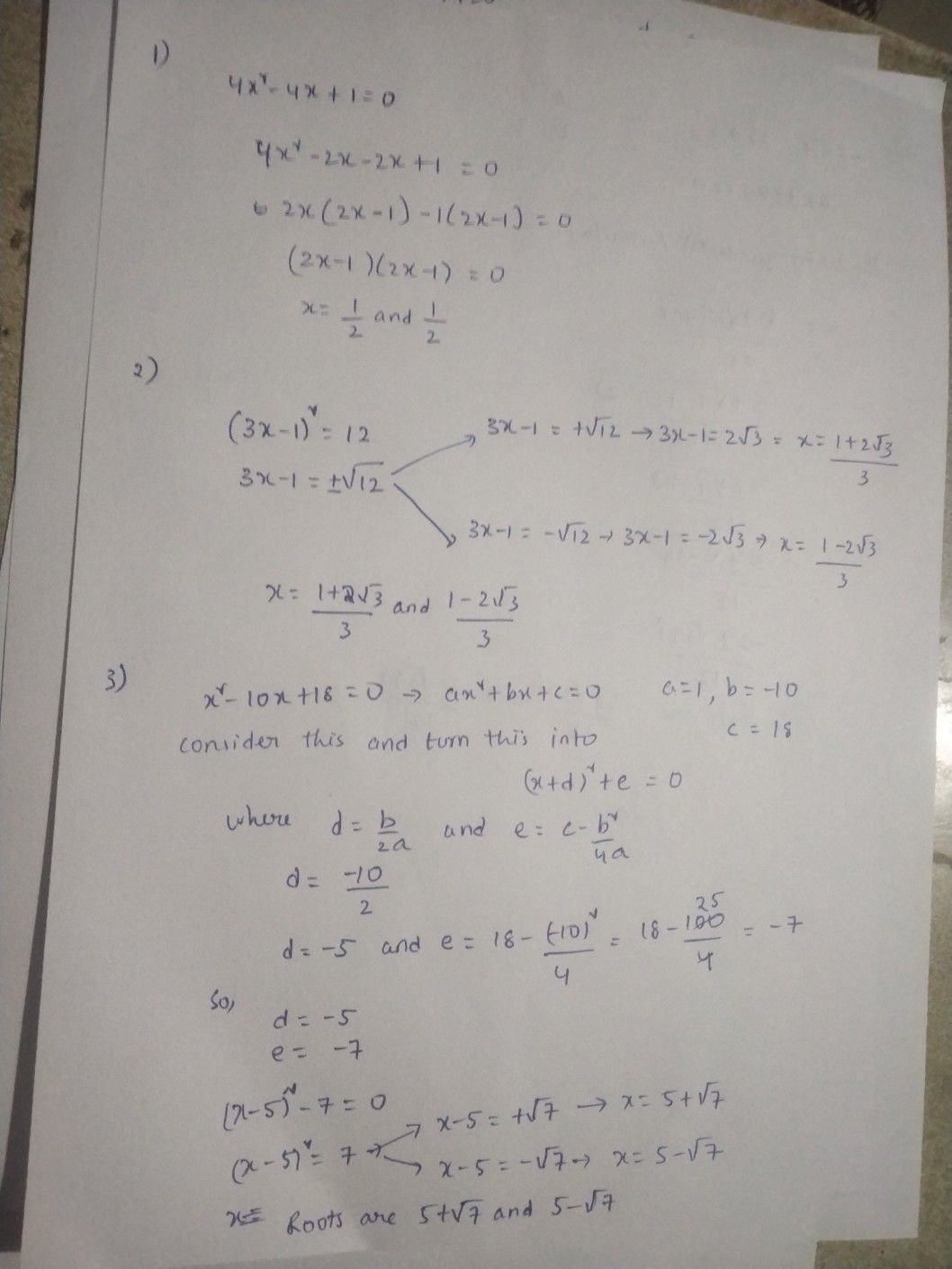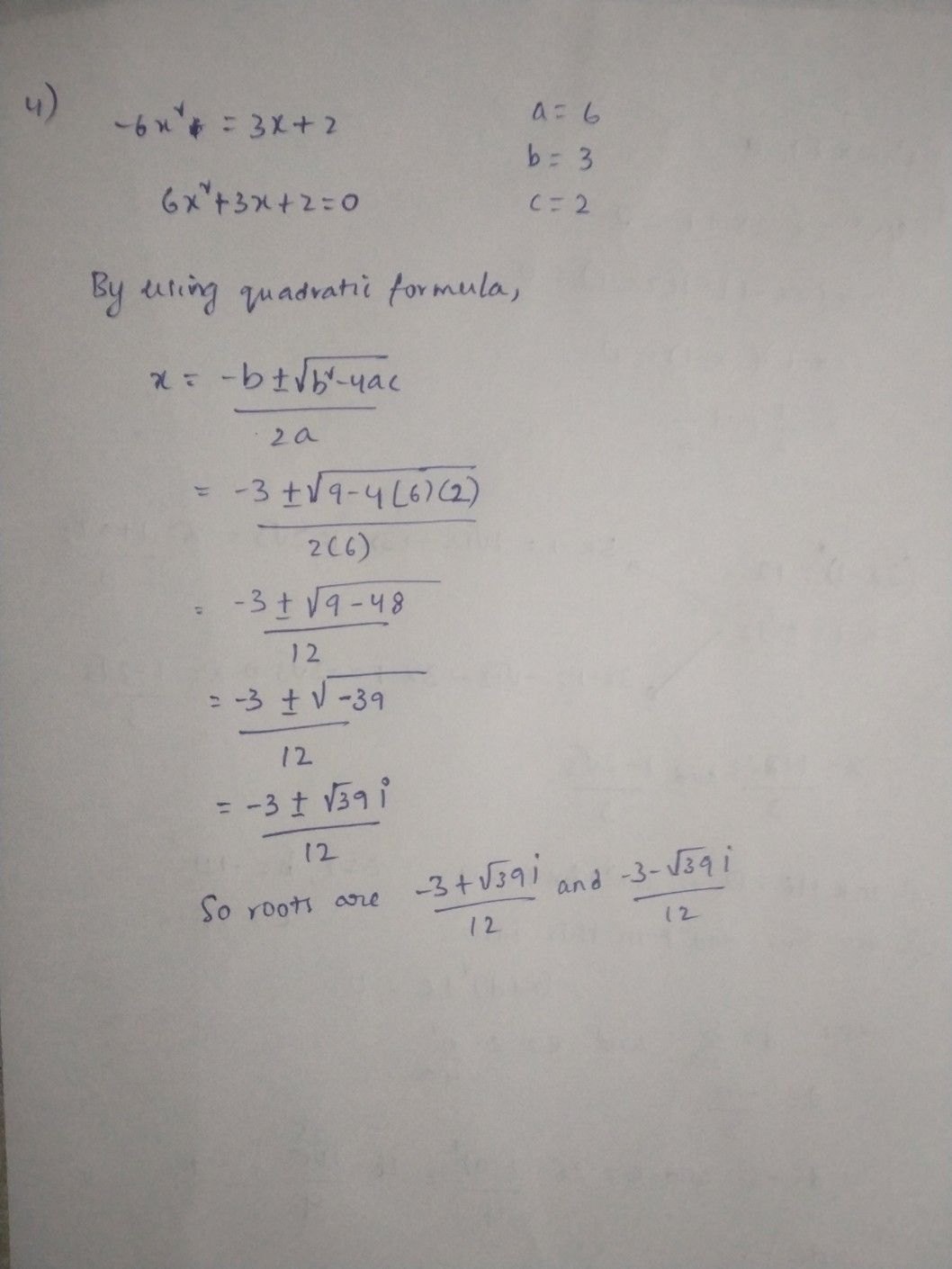Symbol
Problemgrade, which is 20% of your total class grade. Solve each quadratic equation using the suggested method. You must you the suggeste the points. $1$ Solve by factoring. $4x^{2}-4x+1=0$ $2$ Solve by taking the square root. $\left(3x-1$ $\right)^{2}=12$ $3$ Solve by completing the square. $x^{2}-10x+18=0$ Solve by using the quadratic formula. $-6x^{2}=3x+2$ $4.S0$
Algebra
SolutionQanda teacher - saikumarhope it helps
feel free to ask any doubt